# Chemical Equations Reactions http www unit 5 orgchemistryEquations

• Slides: 76Chemical Equations & Reactions http: //www. unit 5. org/chemistry/Equations. html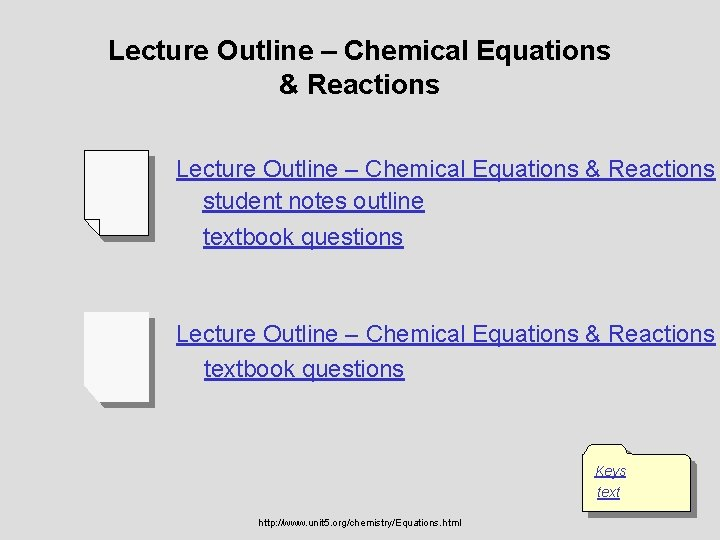Lecture Outline – Chemical Equations & Reactions student notes outline textbook questions Lecture Outline – Chemical Equations & Reactions textbook questions Keys text http: //www. unit 5. org/chemistry/Equations. html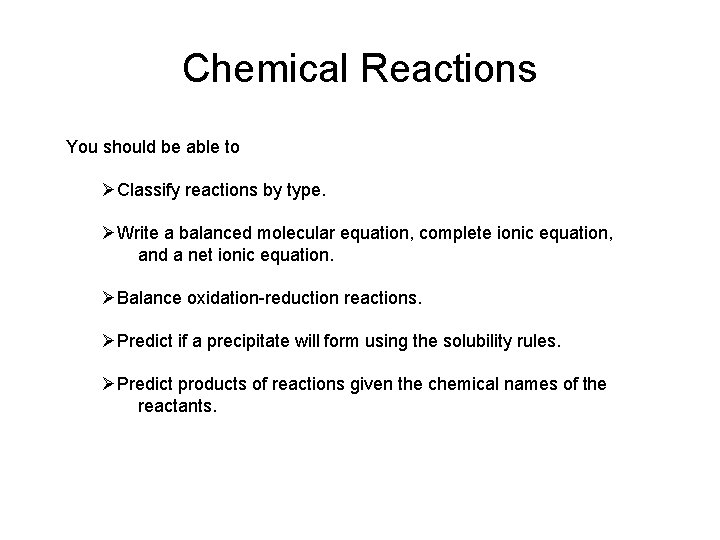Chemical Reactions You should be able to ØClassify reactions by type. ØWrite a balanced molecular equation, complete ionic equation, and a net ionic equation. ØBalance oxidation-reduction reactions. ØPredict if a precipitate will form using the solubility rules. ØPredict products of reactions given the chemical names of the reactants.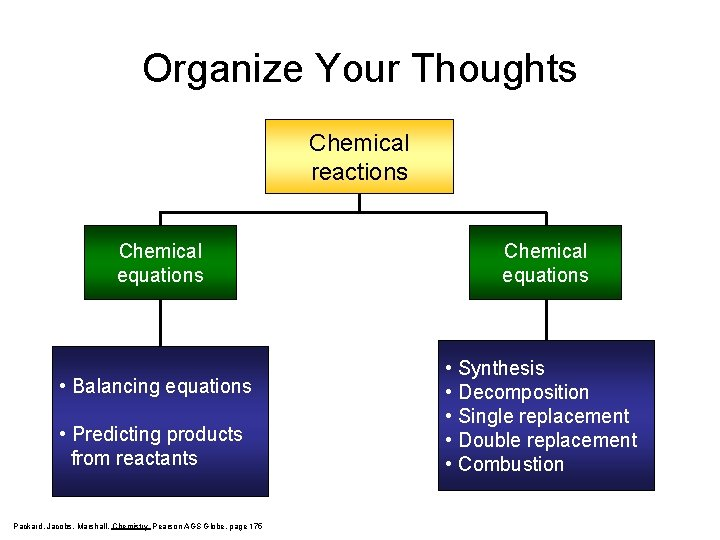Organize Your Thoughts Chemical reactions Chemical equations • Balancing equations • Predicting products from reactants Packard, Jacobs, Marshall, Chemistry Pearson AGS Globe, page 175 Chemical equations • Synthesis • Decomposition • Single replacement • Double replacement • CombustionDescribing a Chemical Reaction Indications of a Chemical Reaction – Evolution of heat, light, and/or sound – Production of a gas – Formation of a precipitate – Color changeSigns of Chemical Reactions There are five main signs that indicate a chemical reaction has taken place: release input change in color change in odor production of new gases or vapor input or release of energy difficult to reverse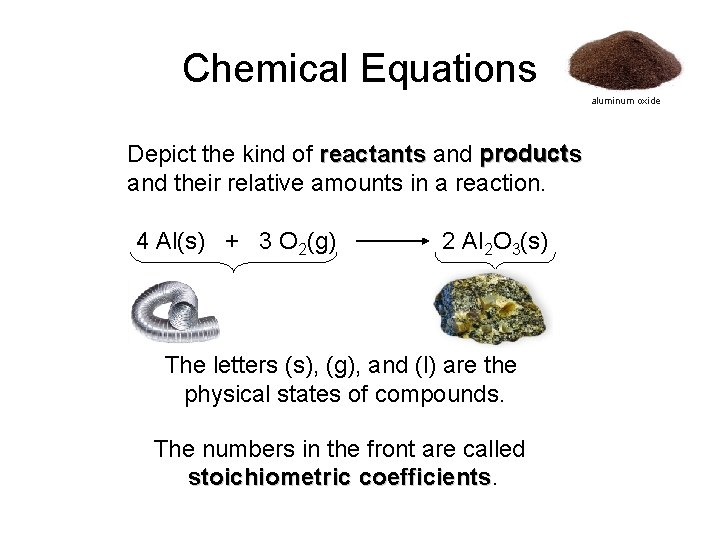Chemical Equations aluminum oxide Depict the kind of reactants and products and their relative amounts in a reaction. 4 Al(s) + 3 O 2(g) 2 Al 2 O 3(s) The letters (s), (g), and (l) are the physical states of compounds. The numbers in the front are called stoichiometric coefficients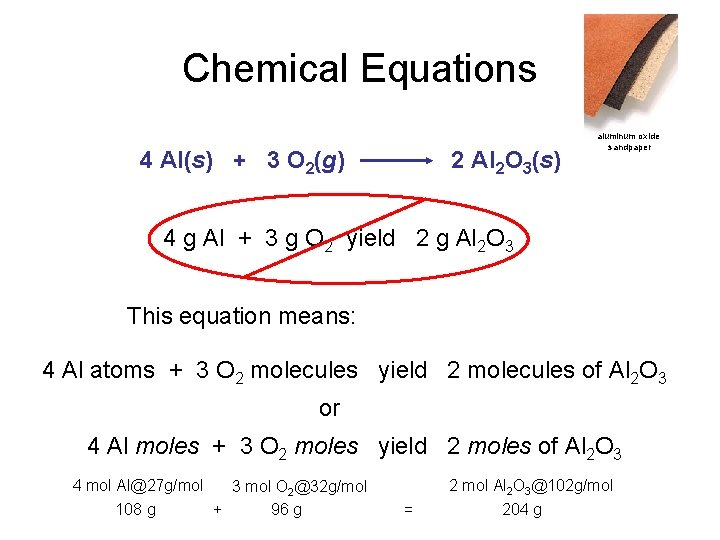Chemical Equations 4 Al(s) + 3 O 2(g) 2 Al 2 O 3(s) aluminum oxide sandpaper 4 g Al + 3 g O 2 yield 2 g Al 2 O 3 This equation means: 4 Al atoms + 3 O 2 molecules yield 2 molecules of Al 2 O 3 or 4 Al moles + 3 O 2 moles yield 2 moles of Al 2 O 3 4 mol [email protected] g/mol 3 mol O [email protected] g/mol 108 g + 96 g 2 mol Al 2 O [email protected] g/mol = 204 gChemical Equations Because the same atoms are present in a reaction at the beginning (reactants) and at the end (products), the amount of matter in a system does not change. The Law of Conservation of Matter Kotz webChemical Equations Because of the principle of the conservation of matter, matter An equation must be balanced It must have the same number of atoms of the same kind on both sides. Lavoisier, 1788Characteristics of Chemical Equations • The equation must represent known facts. • The equation must contain the correct formulas for the reactants and products. • The law of conservation of mass must be satisfied.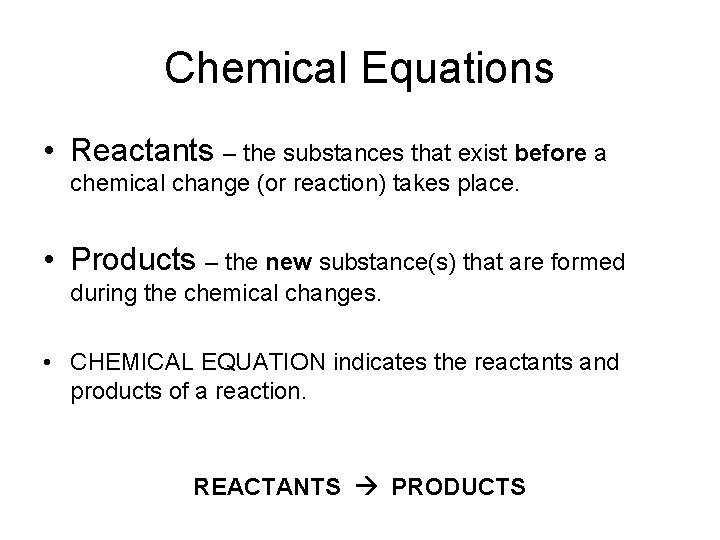Chemical Equations • Reactants – the substances that exist before a chemical change (or reaction) takes place. • Products – the new substance(s) that are formed during the chemical changes. • CHEMICAL EQUATION indicates the reactants and products of a reaction. REACTANTS PRODUCTS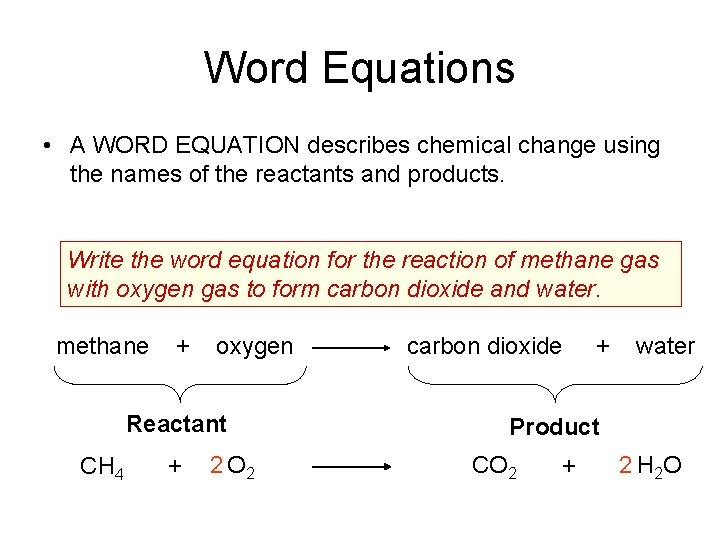Word Equations • A WORD EQUATION describes chemical change using the names of the reactants and products. Write the word equation for the reaction of methane gas with oxygen gas to form carbon dioxide and water. methane + oxygen Reactant CH 4 + 2 O 2 carbon dioxide + water Product CO 2 + 2 H 2 O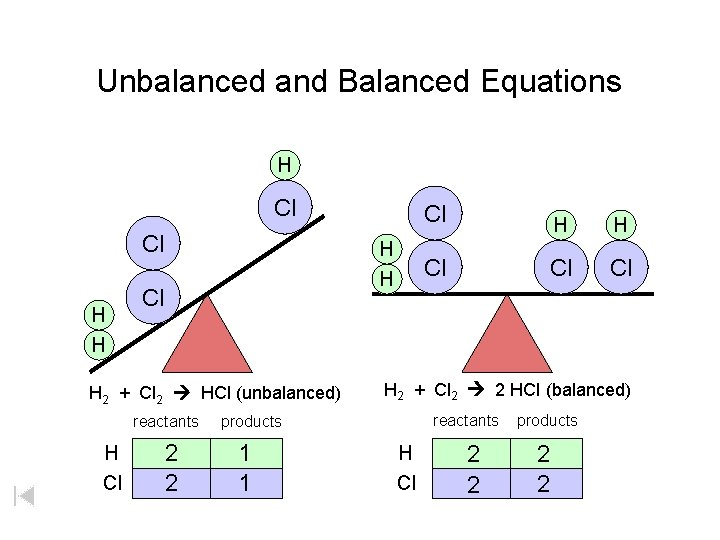Unbalanced and Balanced Equations H Cl Cl H H Cl H 2 + Cl 2 HCl (unbalanced) reactants H Cl 2 2 H H Cl Cl Cl H 2 + Cl 2 2 HCl (balanced) reactants products 1 1 Cl H Cl 2 2 products 2 2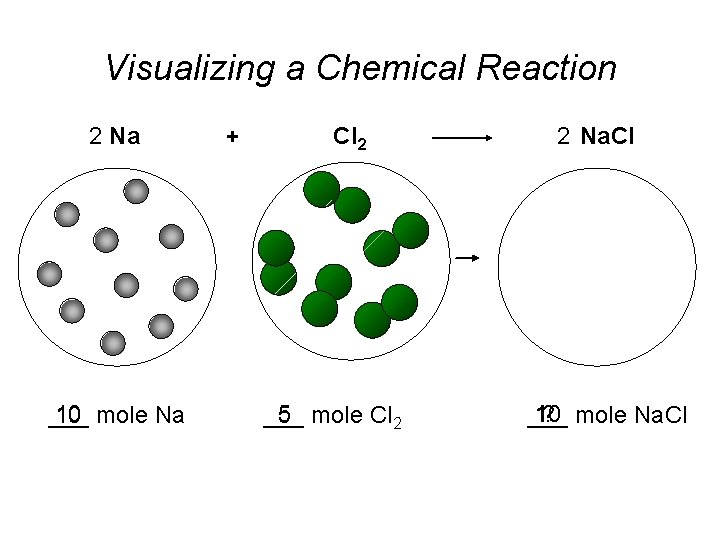Visualizing a Chemical Reaction 2 Na 10 mole Na ___ + Cl 2 5 mole Cl 2 ___ 2 Na. Cl 10 ? mole Na. Cl ___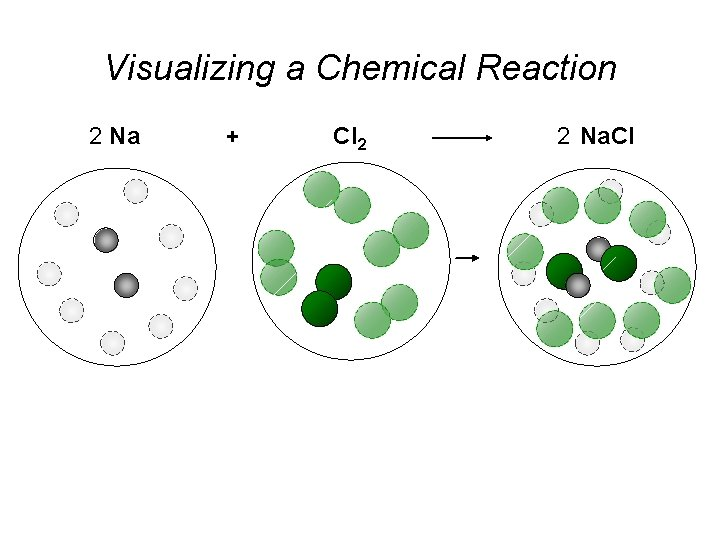Visualizing a Chemical Reaction 2 Na + Cl 2 2 Na. Cl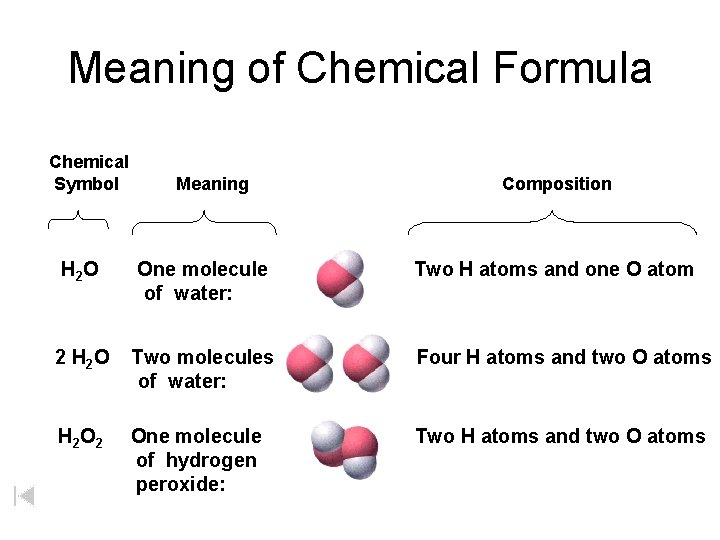Meaning of Chemical Formula Chemical Symbol Meaning Composition H 2 O One molecule of water: Two H atoms and one O atom 2 H 2 O Two molecules of water: Four H atoms and two O atoms H 2 One molecule of hydrogen peroxide: Two H atoms and two O atomsBalancing Chemical Equations Balanced Equation – one in which the number of atoms of each element as a reactant is equal to the number of atoms of that element as a product What is the relationship between conservation of mass and the fact that a balanced equation will always have the same number of atoms of each element on both sides of an equation? Determine whether the following equation is balanced. 2 Na + H 2 O 2 Na. OH + H 2 2 Na + 2 H 2 O 2 Na. OH + H 2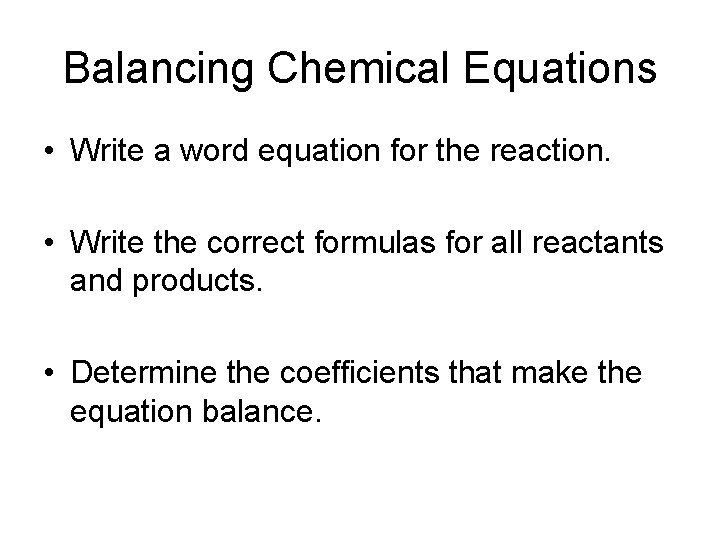Balancing Chemical Equations • Write a word equation for the reaction. • Write the correct formulas for all reactants and products. • Determine the coefficients that make the equation balance.Guidelines for Balancing Chemical Equations ? 1) polyatomic ions first 2) even / odd (make all even) 3) 2 H-OH vs. H 2 O Mg(OH)2 4) single elements last Example: need 13 oxygen atoms “ ” Multiply by 13 O 2 = 13 2 3 X + 13 2 O 2 2 3 X + 6 X + 2 Y + Z O 2 2 Y + Z 13 O 2 4 Y + 2 Z 13 26 NH? 4 OH 2 (NH 4)3 PO 4 + 3 Mg(OH)2 Mg 3(PO 4)2 + ammonium phosphate magnesium hydroxide magnesium phosphate ammonium hydroxide NH 41+ OH 1 - Now you try… 2 Al. Cl 3 + 3 Li 2 CO 3 Al 2(CO 3)3 + 6 Li. Cl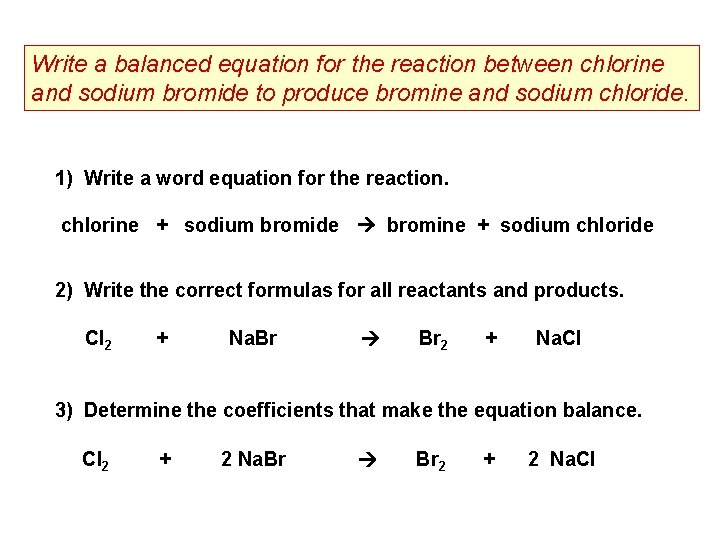Write a balanced equation for the reaction between chlorine and sodium bromide to produce bromine and sodium chloride. 1) Write a word equation for the reaction. chlorine + sodium bromide bromine + sodium chloride 2) Write the correct formulas for all reactants and products. Cl 2 + Na. Br Br 2 + Na. Cl 3) Determine the coefficients that make the equation balance. Cl 2 + 2 Na. Br Br 2 + 2 Na. Cl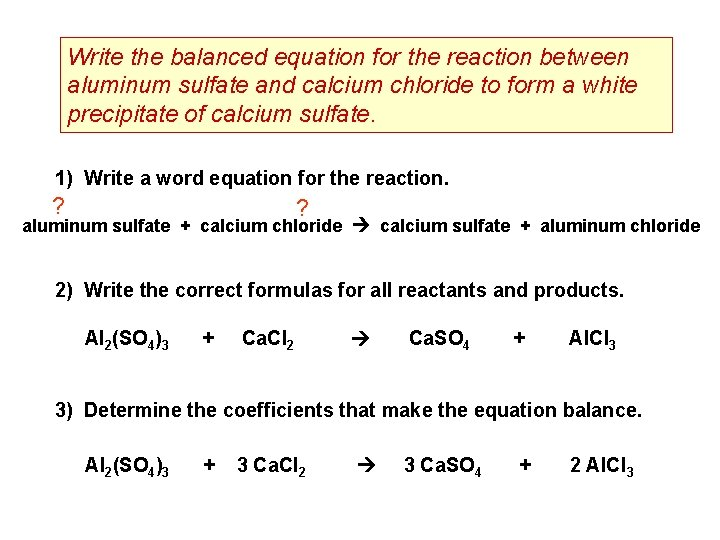Write the balanced equation for the reaction between aluminum sulfate and calcium chloride to form a white precipitate of calcium sulfate. 1) Write a word equation for the reaction. ? ? aluminum sulfate + calcium chloride calcium sulfate + aluminum chloride 2) Write the correct formulas for all reactants and products. Al 2(SO 4)3 + Ca. Cl 2 Ca. SO 4 + Al. Cl 3 3) Determine the coefficients that make the equation balance. Al 2(SO 4)3 + 3 Ca. Cl 2 3 Ca. SO 4 + 2 Al. Cl 3CH 4 + 2 O 2 CO 2 + 2 H 2 O Reactants 1 C atom 4 H atoms 4 O atoms Products 1 C atom 4 H atoms 4 O atomsReactants Products catalyst – speeds up reaction 2 2 Unbalanced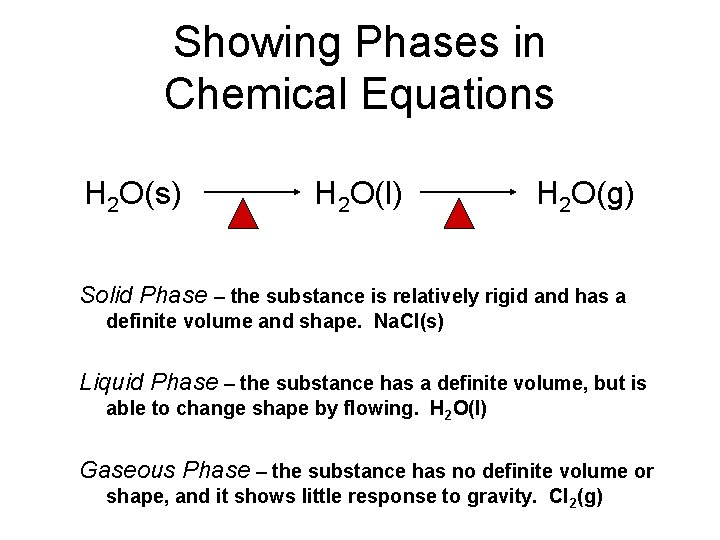Showing Phases in Chemical Equations H 2 O(s) H 2 O(l) H 2 O(g) Solid Phase – the substance is relatively rigid and has a definite volume and shape. Na. Cl(s) Liquid Phase – the substance has a definite volume, but is able to change shape by flowing. H 2 O(l) Gaseous Phase – the substance has no definite volume or shape, and it shows little response to gravity. Cl 2(g)Additional Symbols Used in Chemical Equations “Yields”; indicates result of reaction Used to indicate a reversible reaction (s) A reactant or product in the solid state; also used to indicate a precipitate Alternative to (s), but used only to indicate a precipitate (l) A reactant or product in the liquid state (aq) A reactant or product in an aqueous solution (dissolved in water) (g) A reactant or product in the gaseous state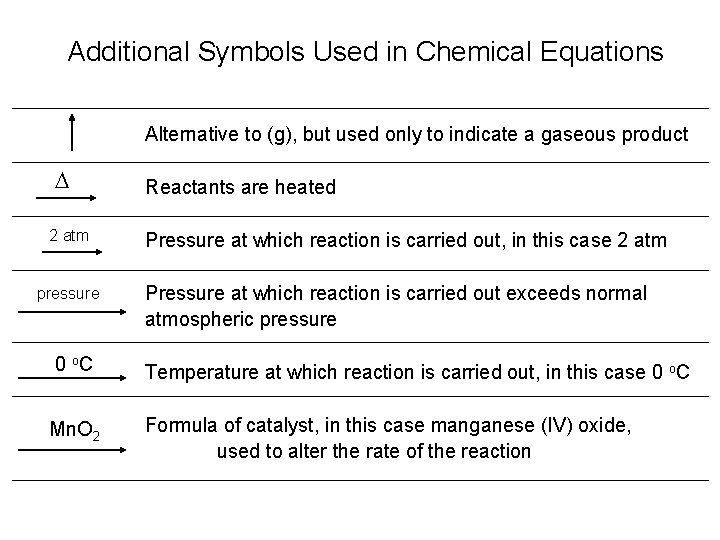Additional Symbols Used in Chemical Equations Alternative to (g), but used only to indicate a gaseous product D 2 atm pressure Reactants are heated Pressure at which reaction is carried out, in this case 2 atm Pressure at which reaction is carried out exceeds normal atmospheric pressure 0 o. C Temperature at which reaction is carried out, in this case 0 o. C Mn. O 2 Formula of catalyst, in this case manganese (IV) oxide, used to alter the rate of the reaction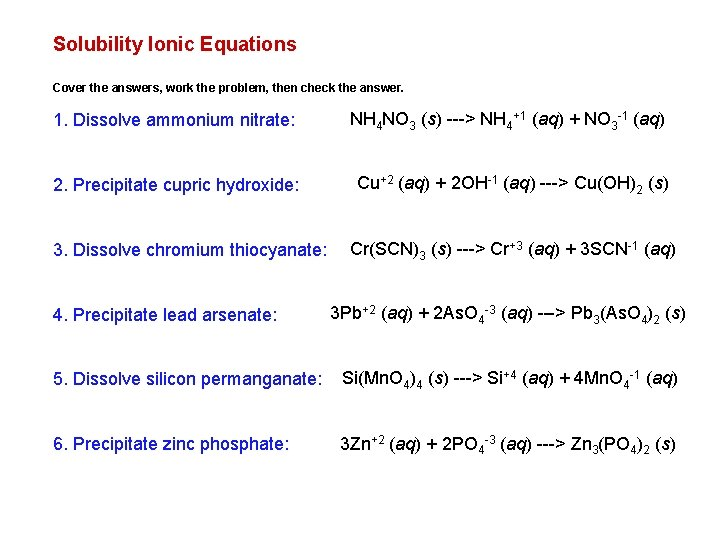Solubility Ionic Equations Cover the answers, work the problem, then check the answer. 1. Dissolve ammonium nitrate: NH 4 NO 3 (s) ---> NH 4+1 (aq) + NO 3 -1 (aq) 2. Precipitate cupric hydroxide: Cu+2 (aq) + 2 OH-1 (aq) ---> Cu(OH)2 (s) 3. Dissolve chromium thiocyanate: 4. Precipitate lead arsenate: Cr(SCN)3 (s) ---> Cr+3 (aq) + 3 SCN-1 (aq) 3 Pb+2 (aq) + 2 As. O 4 -3 (aq) ---> Pb 3(As. O 4)2 (s) 5. Dissolve silicon permanganate: Si(Mn. O 4)4 (s) ---> Si+4 (aq) + 4 Mn. O 4 -1 (aq) 6. Precipitate zinc phosphate: 3 Zn+2 (aq) + 2 PO 4 -3 (aq) ---> Zn 3(PO 4)2 (s)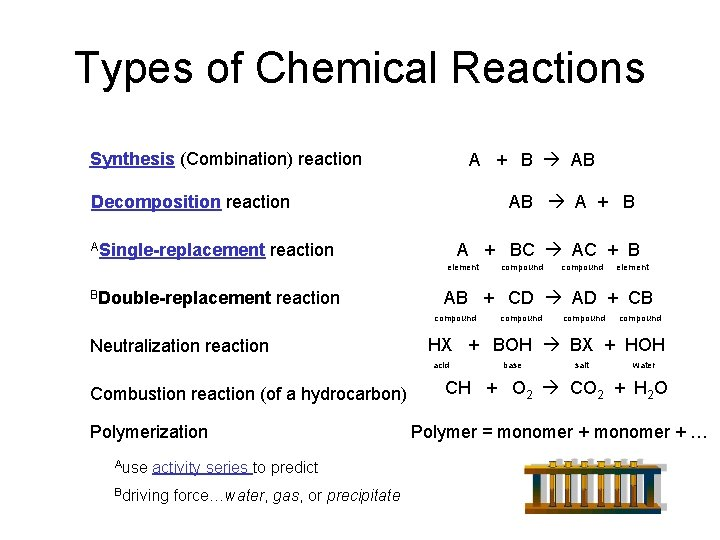Types of Chemical Reactions Synthesis (Combination) reaction A + B AB AB A + B Decomposition reaction ASingle-replacement A + BC AC + B reaction element BDouble-replacement reaction Polymerization Ause activity series to predict Bdriving force…water, gas, or precipitate element compound HX + BOH BX + HOH acid Combustion reaction (of a hydrocarbon) compound AB + CD AD + CB compound Neutralization reaction compound base salt water CH + O 2 CO 2 + H 2 O Polymer = monomer + …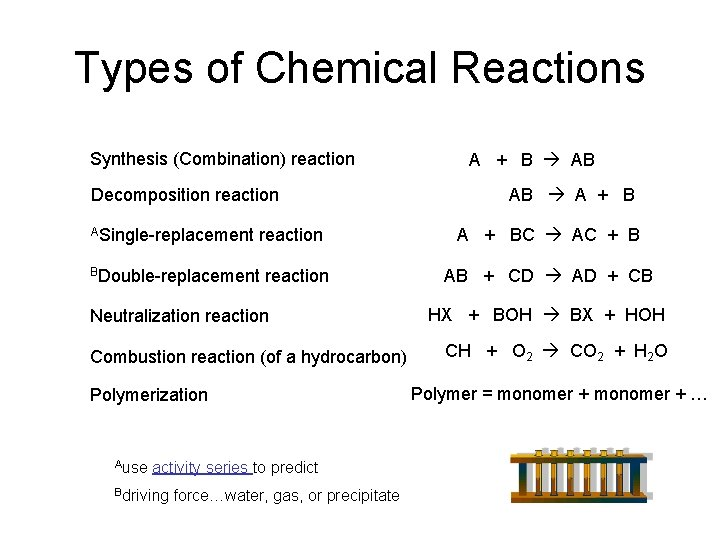Types of Chemical Reactions Synthesis (Combination) reaction Decomposition reaction ASingle-replacement reaction BDouble-replacement reaction Neutralization reaction Combustion reaction (of a hydrocarbon) Polymerization Ause activity series to predict Bdriving force…water, gas, or precipitate A + B AB AB A + BC AC + B AB + CD AD + CB HX + BOH BX + HOH CH + O 2 CO 2 + H 2 O Polymer = monomer + …Chemical Equations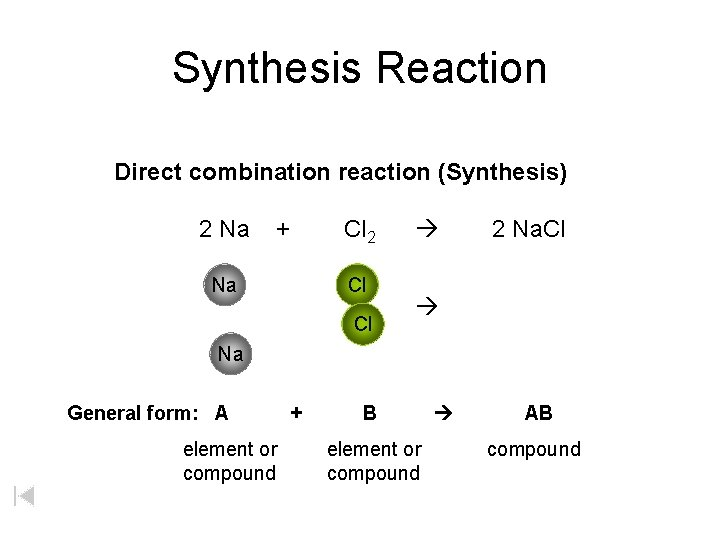Synthesis Reaction Direct combination reaction (Synthesis) 2 Na + Na Cl 2 Cl Cl 2 Na. Cl Na General form: A element or compound + B element or compound AB compoundSynthesis Reaction Direct combination reaction (Synthesis) 2 Na + Cl 2 Na. Cl Cl Na+ Cl - Cl Cl - Na+ Na General form: A element or compound + B element or compound AB compoundFormation of a solid: Ag. Cl Ag. NO 3(aq) + KCl(aq) KNO 3 (aq) + Ag. Cl(s)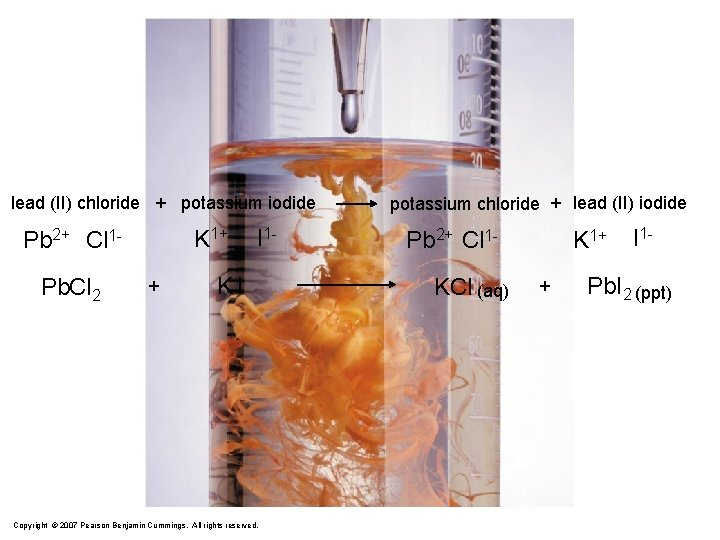lead (II) chloride + potassium iodide K 1+ Pb 2+ Cl 1 Pb. Cl 2 + I 1 - KI Copyright © 2007 Pearson Benjamin Cummings. All rights reserved. potassium chloride + lead (II) iodide Pb 2+ Cl 1 KCl (aq) K 1+ + I 1 - Pb. I 2 (ppt)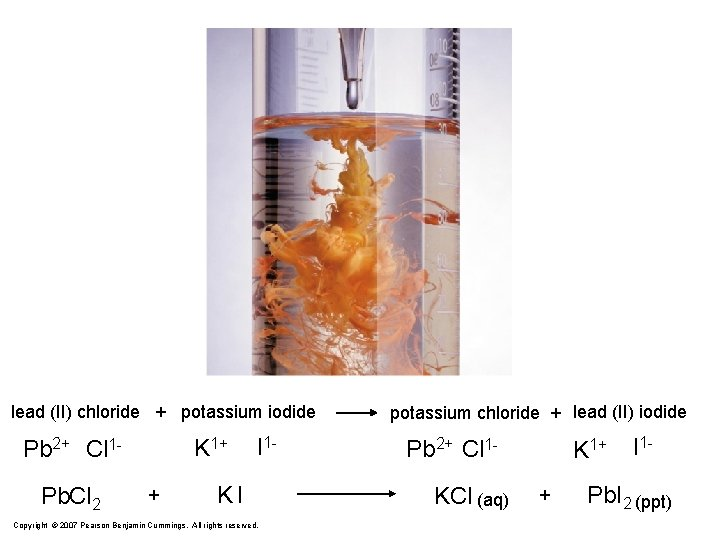lead (II) chloride + potassium iodide K 1+ Pb 2+ Cl 1 Pb. Cl 2 + I 1 - KI Copyright © 2007 Pearson Benjamin Cummings. All rights reserved. potassium chloride + lead (II) iodide Pb 2+ Cl 1 KCl (aq) K 1+ + I 1 - Pb. I 2 (ppt)Decomposition Reaction Decomposition reaction 2 H 2 O 2 H 2 + O 2 H O H + H O H General form: AB compound A + B two or more elements or compounds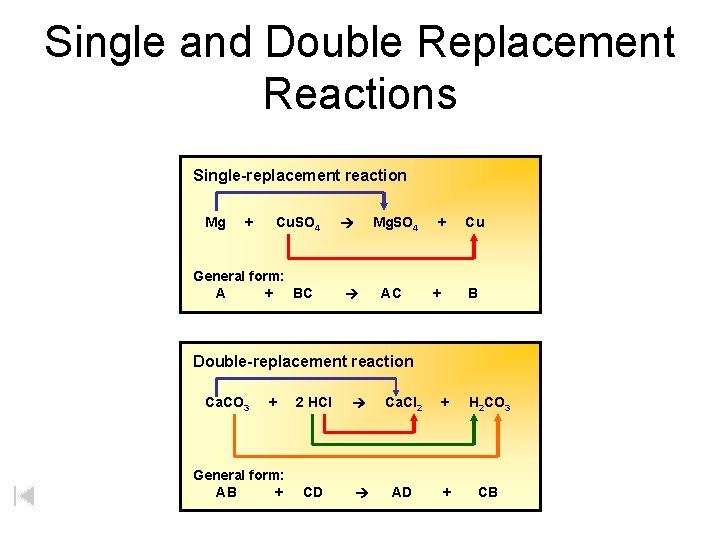Single and Double Replacement Reactions Single-replacement reaction Mg + Cu. SO 4 General form: A + BC Mg. SO 4 AC + + Cu B Double-replacement reaction Ca. CO 3 + General form: AB + 2 HCl Ca. Cl 2 + H 2 CO 3 CD AD + CBPrintable Version of Activity Series Element Reactivity Ca Foiled again – Aluminum loses to Calcium Li Rb K Ba Ca Na Mg Al Mn Zn Cr Fe Ni Sn Pb H 2 Cu Hg Ag Pt Au Halogen Reactivity F 2 Cl 2 Br 2 I 2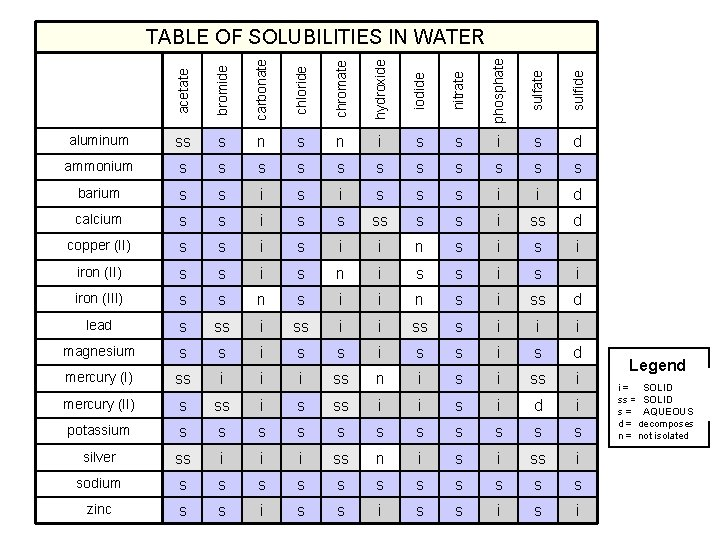acetate bromide carbonate chloride chromate hydroxide iodide nitrate phosphate sulfide TABLE OF SOLUBILITIES IN WATER aluminum ss s n i s s i s d ammonium s s s barium s s i s s s i i d calcium s s i ss d copper (II) s s i i n s i iron (II) s s i s n i s s i iron (III) s s n s i i n s i ss d lead s ss i i ss s i i i magnesium s s i s d mercury (I) ss i i i ss n i ss i mercury (II) s ss i i s i d i potassium s s silver ss i i i ss n i ss i sodium s s s zinc s s i Legend SOLID i = insoluble SOLIDsoluble ss = slightly AQUEOUS s = soluble d = decomposes n = not isolated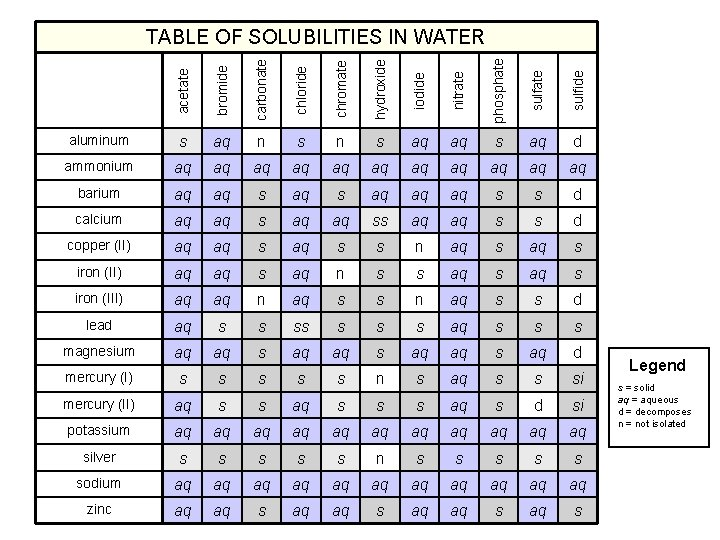acetate bromide carbonate chloride chromate hydroxide iodide nitrate phosphate sulfide TABLE OF SOLUBILITIES IN WATER aluminum s aq n s aq aq s aq d ammonium aq aq aq barium aq aq s aq aq aq s s d calcium aq aq ss aq aq s s d copper (II) aq aq s s n aq s iron (II) aq aq s aq n s s aq s iron (III) aq aq n aq s s d lead aq s s s s aq s s s magnesium aq aq s aq d mercury (I) s s s n s aq s s si mercury (II) aq s s s aq s d si potassium aq aq aq silver s s s n s s sodium aq aq aq zinc aq aq s Legend s = solid aq = aqueous d = decomposes n = not isolatedacetate bromide carbonate chloride chromate hydroxide iodide nitrate phosphate sulfide TABLE OF SOLUBILITIES IN WATER aluminum ss s n i s s i s d ammonium s s s barium s s i s s s i i d calcium s s i ss d copper (II) s s i i n s i iron (II) s s i s n i s s i iron (III) s s n s i i n s i ss d lead s ss i i ss s i i i magnesium s s i s d mercury (I) ss i i i ss n i ss i mercury (II) s ss i i s i d i potassium s s silver ss i i i ss n i ss i sodium s s s zinc s s i Legend s = solid aq = aqueous d = decomposes n = not isolated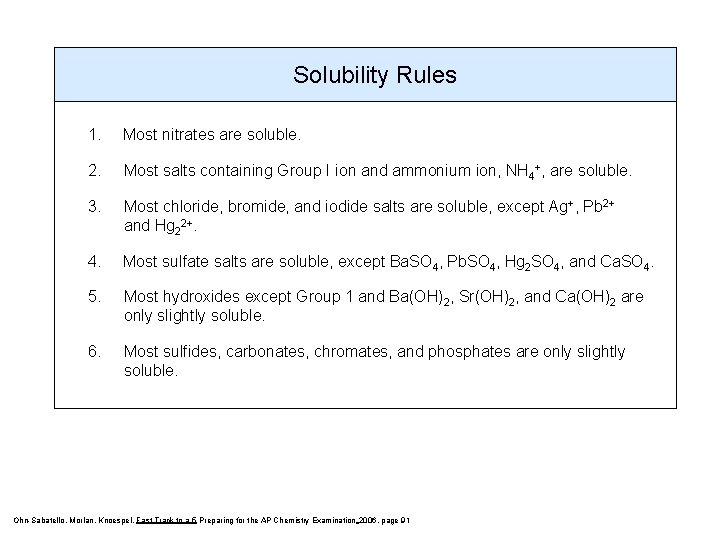Solubility Rules 1. Most nitrates are soluble. 2. Most salts containing Group I ion and ammonium ion, NH 4+, are soluble. 3. Most chloride, bromide, and iodide salts are soluble, except Ag+, Pb 2+ and Hg 22+. 4. Most sulfate salts are soluble, except Ba. SO 4, Pb. SO 4, Hg 2 SO 4, and Ca. SO 4. 5. Most hydroxides except Group 1 and Ba(OH)2, Sr(OH)2, and Ca(OH)2 are only slightly soluble. 6. Most sulfides, carbonates, chromates, and phosphates are only slightly soluble. Ohn-Sabatello, Morlan, Knoespel, Fast Track to a 5 Preparing for the AP Chemistry Examination 2006, page 91Potassium reacts with Water POW!Double Replacement Reaction K 2 CO 3 (aq) Potassium carbonate + Ba. Cl 2 (aq) Barium chloride 2 KCl (aq) Potassium chloride + Ba. CO 3 (s) Barium carbonate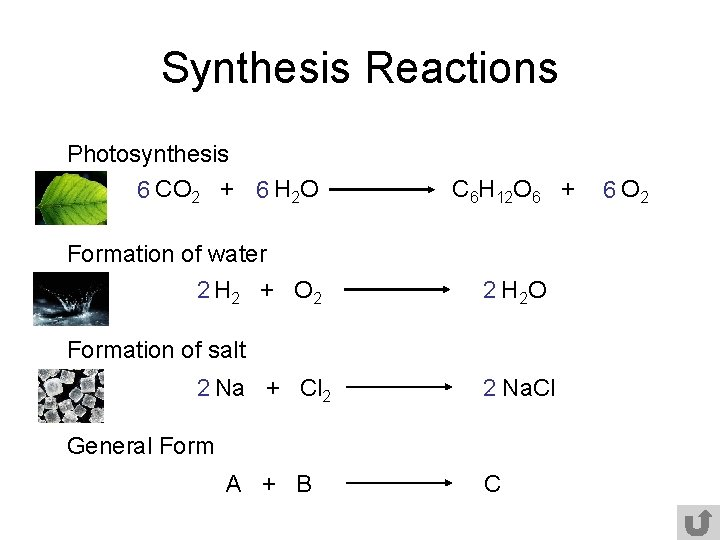Synthesis Reactions Photosynthesis 6 CO 2 + 6 H 2 O C 6 H 12 O 6 + Formation of water 2 H 2 + O 2 2 H 2 O Formation of salt 2 Na + Cl 2 2 Na. Cl General Form A + B C 6 O 2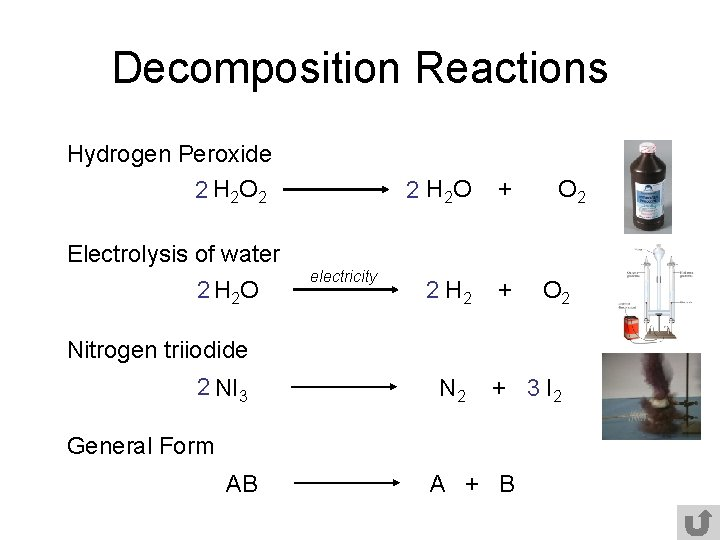Decomposition Reactions Hydrogen Peroxide 2 H 2 O 2 2 H 2 O + 2 H 2 + O 2 Electrolysis of water 2 H 2 O electricity O 2 Nitrogen triiodide 2 NI 3 N 2 + 3 I 2 General Form AB A + BPredict if these reactions will occur 3 Mg + 2 Al. Cl 3 2 Al + 3 Mg. Cl 2 Can magnesium replace aluminum? YES, magnesium is more reactive than aluminum. Activity Series Al + Mg. Cl 2 No reaction Can aluminum replace magnesium? NO, aluminum is less reactive than magnesium. Activity Series Mg. Cl 2 + Al Therefore, no reaction will occur. No reaction Order of reactants DOES NOT determine how they react. The question we must ask is can the single element replace its counterpart? metal replaces metal or nonmetal replaces nonmetal.Single-Replacement Reactions Activity Series “Magic blue-earth” Fe + Cu. Cl 2 Fe. Cl 2 + Cu Can Fe replace Cu? Yes Zinc in nitric acid Zn + 2 HNO 3 Can Zn replace H? Zn(NO 3)2 + H 2 Yes NO REACTION Mg. Cl 2 + Can Br replace Cl? Br 2 Mg. Br 2 + No General Form A + BC AC + B Cl 2 Li Rb K Ba Ca Na Mg Al Mn Zn Cr Fe Ni Sn Pb H 2 Cu Hg Ag Pt Au F 2 Cl 2 Br 2 I 2How would you prepare potassium nitrate (using a double replacement reaction)? KKOH NO 33 HHNO OH ++ _________ Ca(NO 3)2 2 KOH + Ca(NO 3)2 formation of water is a driving force. KNO 33 ++ _____ H 22 O O KNO H Both potassium nitrate and calcium chloride are soluble (no driving force – no reaction!) 2 KNO 3 + Ca(OH)2 Combine a potassium hydroxide solution with nitric acid to yield soluble potassium nitrate. KOH(aq) + HNO 3(aq) KNO 3(aq) + H? 2 O The water could then be removed by distillation to recover solid potassium nitrate.Predict if a reaction will occur when you combine aqueous solutions of iron (II) chloride with aqueous sodium carbonate solution. If the reaction does occur, write a Balanced balanced chemical equation showing it. (be sure to include phase notation) iron (II) chloride + sodium carbonate Fe 2+ Cl 1 - Na 1+ CO 32 - Fe. Cl 2 Na 2 CO 3 sodium chloride + iron (II) carbonate Na 1+ CO 32 - Fe 2+ Cl 1 - Fe. CO 3 (ppt) Na. Cl (aq) Using a SOLUBILITY TABLE: sodium chloride is soluble iron (II) carbonate is insoluble Fe. Cl 2 (aq) + Na 2 CO 3 (aq) Complete Ionic Equation Fe 2+(aq) + 2 Cl 1 -(aq) + 2 Na 1+(aq) + CO 32 -(aq) 2 Na. Cl (aq) + Fe. CO 3 (ppt) 2 Na 1+(aq) + 2 Cl 1 -(aq) + Fe. CO 3(s)Visualizing a Chemical Reaction 2 Na 10 mole Na ___ + Cl 2 5 mole Cl 2 ___ 2 Na. Cl 10 ? mole Na. Cl ___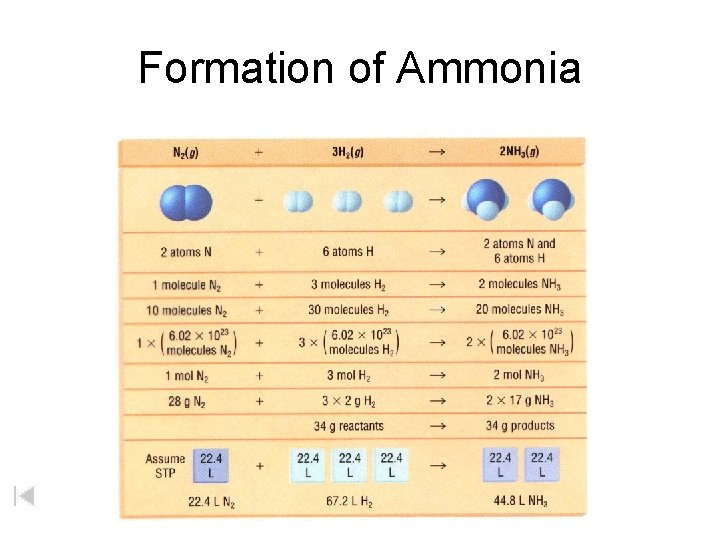Formation of AmmoniaProportional Relationships 2 1/4 c. flour 1 tsp. baking soda 1 tsp. salt Conversion Factor 1 c. butter 3/4 c. sugar 3/4 c. brown sugar 1 tsp vanilla extract 2 eggs 2 c. chocolate chips Makes 5 dozen cookies. I have 5 eggs. How many cookies can I make? 5 eggs 5 dozen 2 eggs Ratio of eggs to cookies 150 dozen cookies = 12. 5 Courtesy Christy Johannesson www. nisd. net/communicationsarts/pages/chem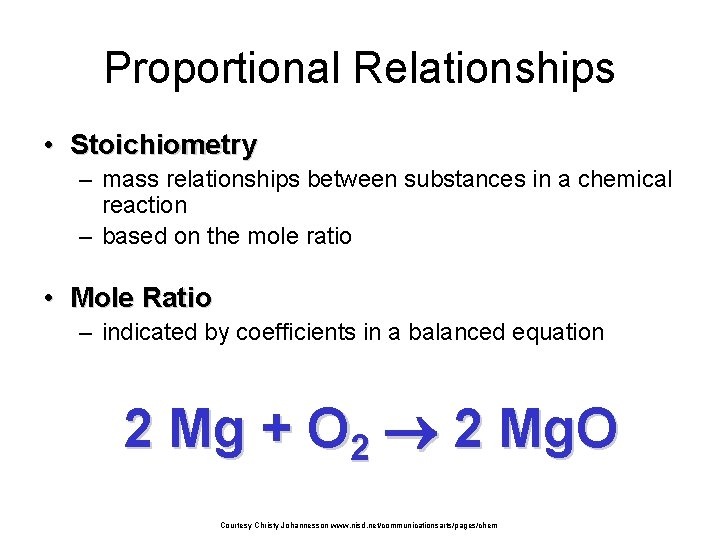Proportional Relationships • Stoichiometry – mass relationships between substances in a chemical reaction – based on the mole ratio • Mole Ratio – indicated by coefficients in a balanced equation 2 Mg + O 2 2 Mg. O Courtesy Christy Johannesson www. nisd. net/communicationsarts/pages/chem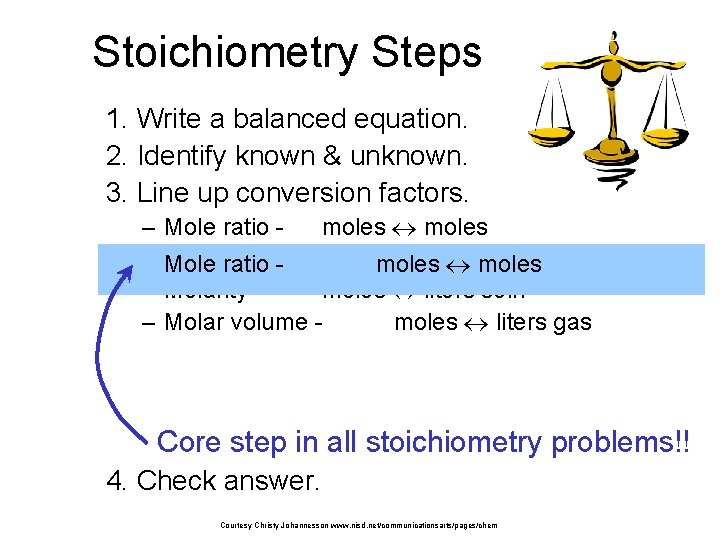Stoichiometry Steps 1. Write a balanced equation. 2. Identify known & unknown. 3. Line up conversion factors. – – – Mole ratio moles Molarratio mass grams Mole - - moles Molarity moles liters soln Molar volume moles liters gas Core step in all stoichiometry problems!! 4. Check answer. Courtesy Christy Johannesson www. nisd. net/communicationsarts/pages/chem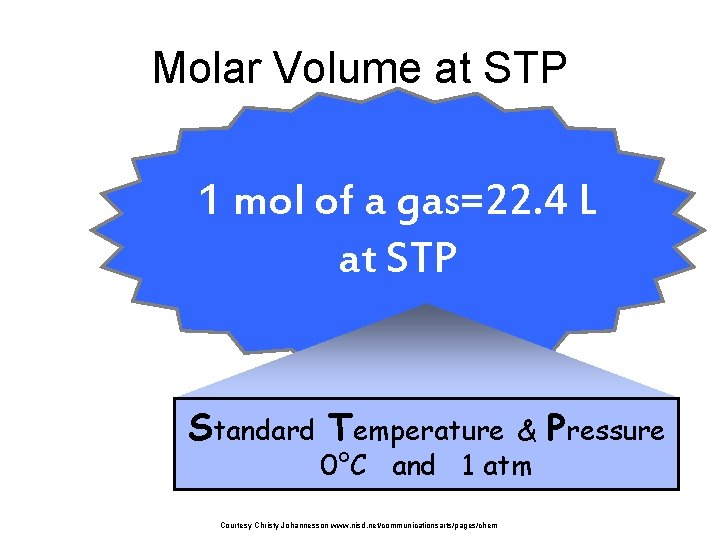Molar Volume at STP 1 mol of a gas=22. 4 L at STP Standard Temperature & 0°C and 1 atm Courtesy Christy Johannesson www. nisd. net/communicationsarts/pages/chem Pressure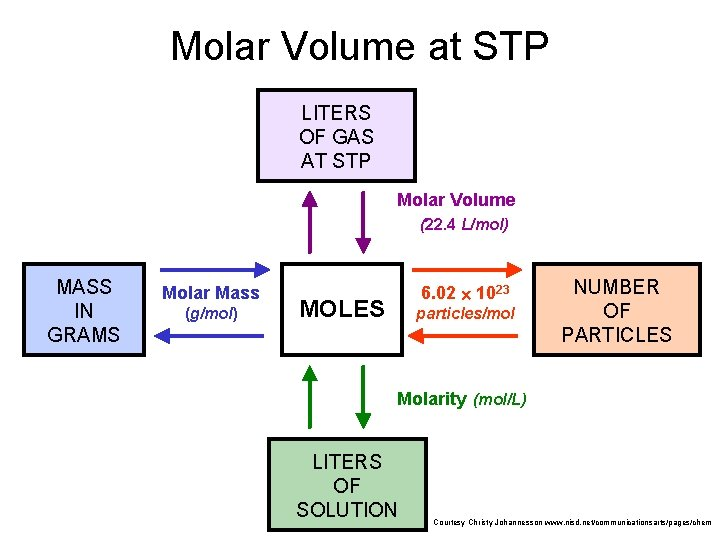Molar Volume at STP LITERS OF GAS AT STP Molar Volume (22. 4 L/mol) MASS IN GRAMS Molar Mass (g/mol) 6. 02 1023 MOLES particles/mol NUMBER OF PARTICLES Molarity (mol/L) LITERS OF SOLUTION Courtesy Christy Johannesson www. nisd. net/communicationsarts/pages/chem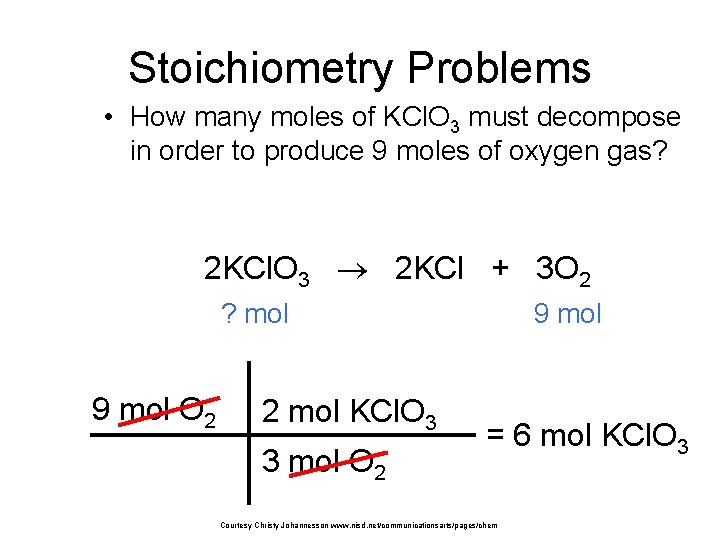Stoichiometry Problems • How many moles of KCl. O 3 must decompose in order to produce 9 moles of oxygen gas? 2 KCl. O 3 2 KCl + 3 O 2 ? mol 9 mol O 2 2 mol KCl. O 3 3 mol O 2 9 mol = 6 mol KCl. O 3 Courtesy Christy Johannesson www. nisd. net/communicationsarts/pages/chem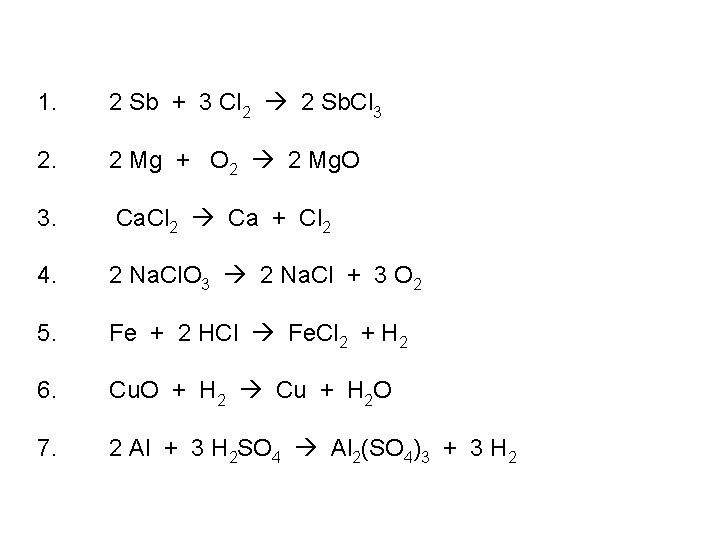1. 2 Sb + 3 Cl 2 2 Sb. Cl 3 2. 2 Mg + O 2 2 Mg. O 3. Ca. Cl 2 Ca + Cl 2 4. 2 Na. Cl. O 3 2 Na. Cl + 3 O 2 5. Fe + 2 HCl Fe. Cl 2 + H 2 6. Cu. O + H 2 Cu + H 2 O 7. 2 Al + 3 H 2 SO 4 Al 2(SO 4)3 + 3 H 2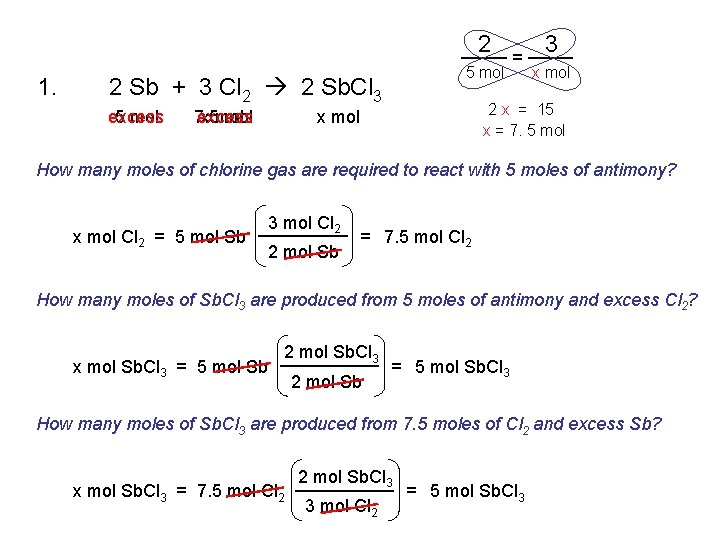2 1. 5 mol 2 Sb + 3 Cl 2 2 Sb. Cl 3 excess 5 mol 7. 5 excess x mol = 3 x mol 2 x = 15 x = 7. 5 mol x mol How many moles of chlorine gas are required to react with 5 moles of antimony? x mol Cl 2 = 5 mol Sb 3 mol Cl 2 2 mol Sb = 7. 5 mol Cl 2 How many moles of Sb. Cl 3 are produced from 5 moles of antimony and excess Cl 2? x mol Sb. Cl 3 = 5 mol Sb 2 mol Sb. Cl 3 2 mol Sb = 5 mol Sb. Cl 3 How many moles of Sb. Cl 3 are produced from 7. 5 moles of Cl 2 and excess Sb? x mol Sb. Cl 3 = 7. 5 mol Cl 2 2 mol Sb. Cl 3 3 mol Cl 2 = 5 mol Sb. Cl 32. 2 Mg + O 2 2 Mg. O 10 mol x. L x mol How many moles of magnesium oxide are produced from the burning of 10 mol of Mg? x mol Mg. O = 10 mol Mg 2 mol Mg. O 2 mol Mg = 10 mol Mg. O How many liters of oxygen are needed to burn 10 mol of Mg? Assume 1 mol O 2 = 22. 4 L x L O 2 = 10 mol Mg 1 mol O 2 2 mol Mg = 5 mol O 2 1 mol O 2 22. 4 L O 2 2 mol Mg 1 mol O 2 22. 4 L O 2 1 mol O 2 = 112 L O 2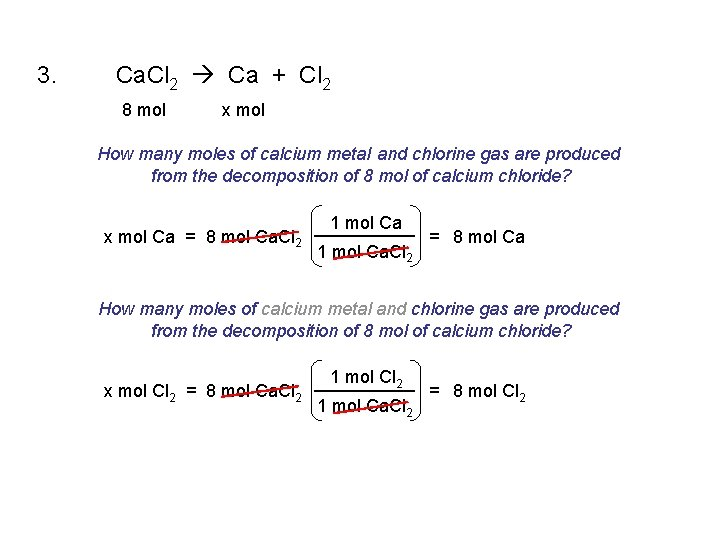3. Ca. Cl 2 Ca + Cl 2 8 mol x mol How many moles of calcium metal and chlorine gas are produced from the decomposition of 8 mol of calcium chloride? x mol Ca = 8 mol Ca. Cl 2 1 mol Ca. Cl 2 = 8 mol Ca How many moles of calcium metal and chlorine gas are produced from the decomposition of 8 mol of calcium chloride? x mol Cl 2 = 8 mol Ca. Cl 2 1 mol Ca. Cl 2 = 8 mol Cl 2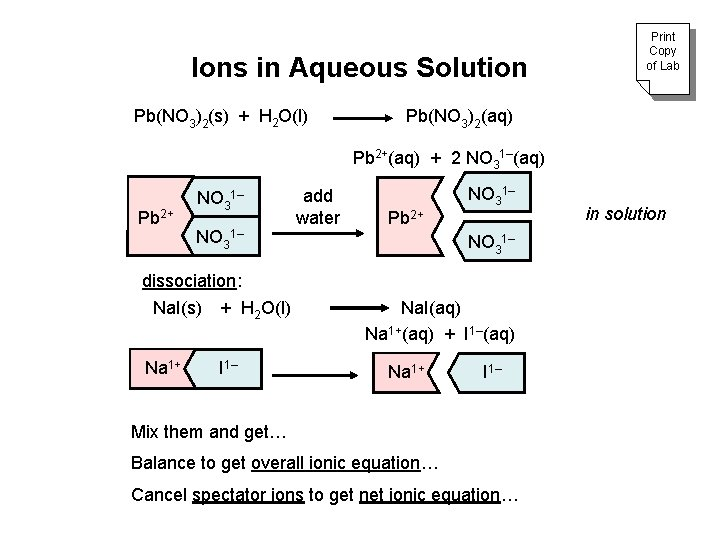Ions in Aqueous Solution Pb(NO 3)2(s) + H 2 O(l) Print Copy of Lab Pb(NO 3)2(aq) Pb 2+(aq) + 2 NO 31–(aq) Pb 2+ NO 31– dissociation: Na. I(s) + H 2 O(l) Na 1+ I 1– add water Pb 2+ NO 31– Na. I(aq) Na 1+(aq) + I 1–(aq) Na 1+ I 1– Mix them and get… Balance to get overall ionic equation… Cancel spectator ions to get net ionic equation… in solution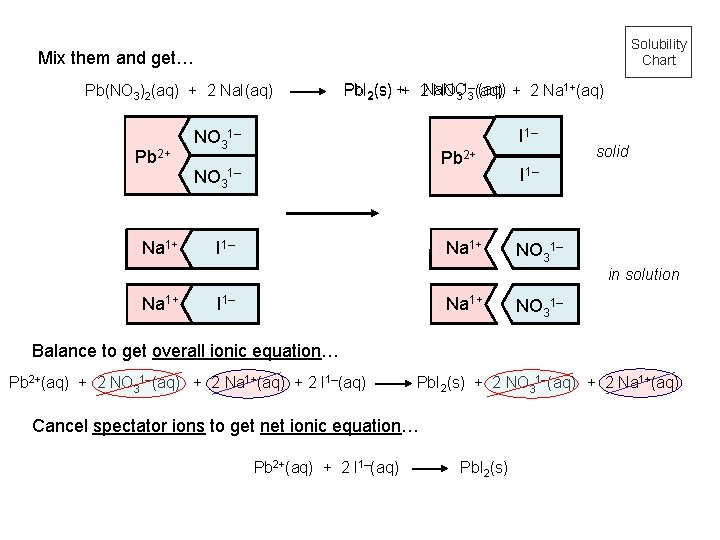Solubility Chart Mix them and get… Pb(NO 3)2(aq) + 2 Na. I(aq) Pb 2+ Na 1+ Pb. I 2(s) ++ 2 Na. NO (aq) + 2 Na 1+(aq) NO 31– 3 (aq) I 1– NO 31– Pb 2+ NO 31– I 1– Na 1+ solid I 1– NO 31– in solution Na 1+ I 1– Na 1+ NO 31– Balance to get overall ionic equation… Pb 2+(aq) + 2 NO 31–(aq) + 2 Na 1+(aq) + 2 I 1–(aq) Pb. I 2(s) + 2 NO 31–(aq) + 2 Na 1+(aq) Cancel spectator ions to get net ionic equation… Pb 2+(aq) + 2 I 1–(aq) Pb. I 2(s)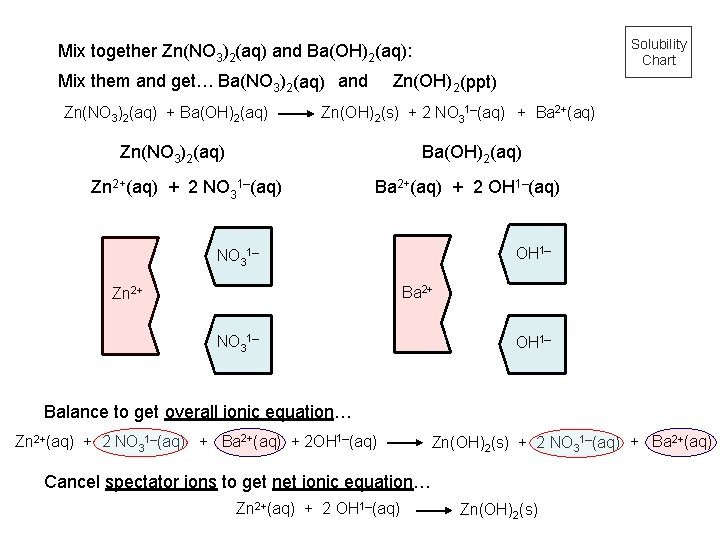Solubility Chart Mix together Zn(NO 3)2(aq) and Ba(OH)2(aq): Mix them and get… Ba(NO 3)2(aq) and Zn(NO 3)2(aq) + Ba(OH)2(aq) Zn(OH)2 (ppt) Zn(OH)2(s) + 2 NO 31–(aq) + Ba 2+(aq) Zn(NO 3)2(aq) Ba(OH)2(aq) Zn 2+(aq) + 2 NO 31–(aq) Ba 2+(aq) + 2 OH 1–(aq) OH 1– NO 31– Ba 2+ Zn 2+ NO 31– OH 1– Balance to get overall ionic equation… Zn 2+(aq) + 2 NO 31–(aq) + Ba 2+(aq) + 2 OH 1–(aq) Zn(OH)2(s) + 2 NO 31–(aq) + Ba 2+(aq) Cancel spectator ions to get net ionic equation… Zn 2+(aq) + 2 OH 1–(aq) Zn(OH)2(s)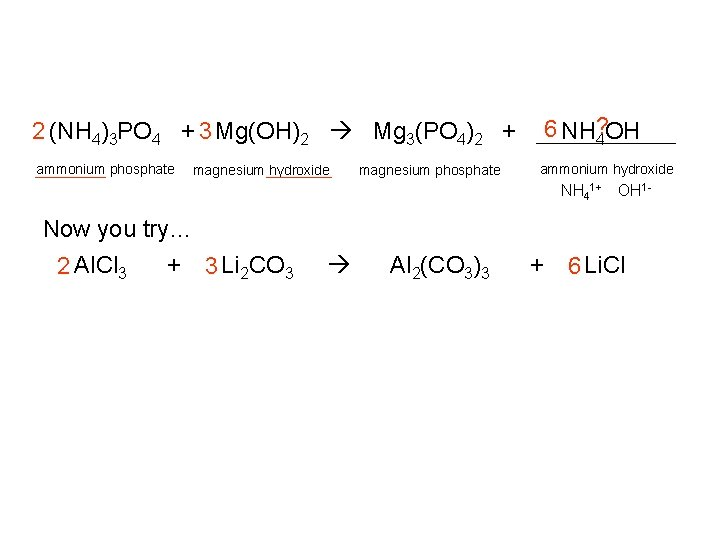6 NH? 4 OH 2 (NH 4)3 PO 4 + 3 Mg(OH)2 Mg 3(PO 4)2 + ammonium phosphate magnesium hydroxide magnesium phosphate ammonium hydroxide NH 41+ OH 1 - Now you try… 2 Al. Cl 3 + 3 Li 2 CO 3 Al 2(CO 3)3 + 6 Li. ClIdentify the spectator ions and write a net ionic equation when an aqueous solution of aluminum sulfate is mixed with aqueous ammonium hydroxide. Al 3+ SO 42 - aluminum sulfate + NH 41+ OH 1 - Al 3+ OH 1 - ammoniumhydroxide aluminum hydroxide + NH 41+ SO 42 - ammonium sulfate Al 2(SO 4)3(aq) + 6 NH 4 OH(aq) 2 Al(OH)3(ppt) + 3 (NH 4)2 SO 4 (aq) 2 Al 3+(aq) + 3 SO 42 -(aq) + 6 NH 41+(aq) + 6 OH 1 -(aq) 2 Al(OH)3(ppt) + 6 NH 41+(aq) + 3 SO 42 -(aq) “spectator ions” 2 Al 3+(aq) + 6 OH 1 -(aq) 2 Al(OH)3(ppt) Net Ionic EquationMeaning of Coefficients 2 atoms Na 1 molecule Cl 2 2 molecules Na. Cl 2 Na + Cl 2 2 g sodium + 1 g chlorine 2 mol sodium (2 mol Na) x (23 g/mol) 2 Na. Cl = 1 mol chlorine (1 mol Cl 2) x (71 g/mol) 46 g 71 g 117 g 2 g sodium chloride 2 mol sodium chloride (2 mol Na. Cl) x (58. 5 g/mol) 117 gClasses of Reactions Chemical reactions Precipitation reactions Oxidation-Reduction Reactions Combustion Reactions Acid-Base Reactions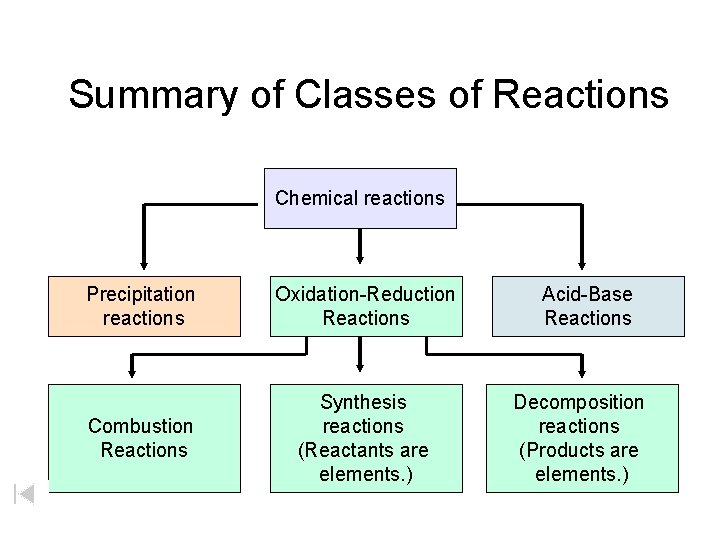Summary of Classes of Reactions Chemical reactions Precipitation reactions Oxidation-Reduction Reactions Combustion Reactions Synthesis reactions (Reactants are elements. ) Acid-Base Reactions Decomposition reactions (Products are elements. )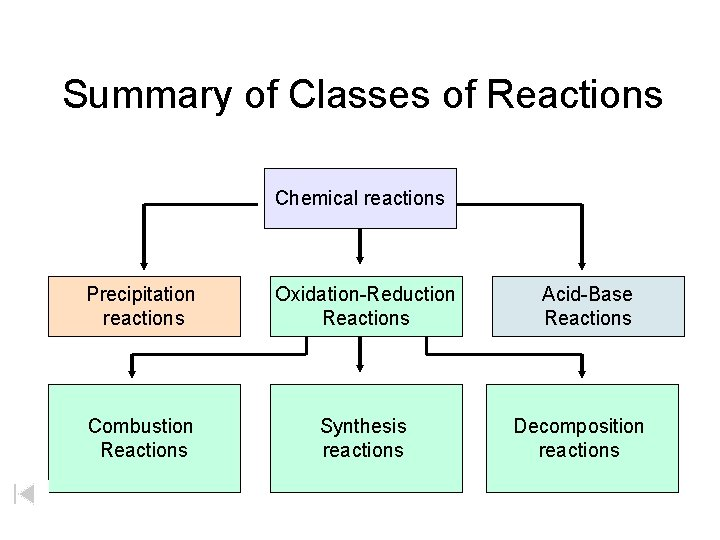Summary of Classes of Reactions Chemical reactions Precipitation reactions Oxidation-Reduction Reactions Combustion Reactions Synthesis reactions Acid-Base Reactions Decomposition reactions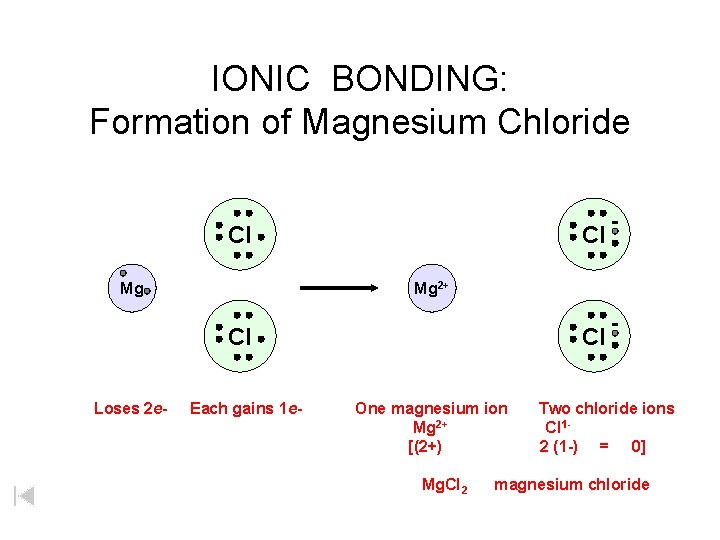IONIC BONDING: Formation of Magnesium Chloride Cl Mg 2+ Cl Loses 2 e- Each gains 1 e- Cl One magnesium ion Mg 2+ [(2+) Mg. Cl 2 Two chloride ions Cl 12 (1 -) = 0] magnesium chlorideIONIC BONDING: Formation of Magnesium Chloride Cl Mg Mg 2+ Cl Loses 2 e- Each gains 1 e- Cl One magnesium ion Mg 2+ [(2+) Mg. Cl 2 Two chloride ions Cl 12 (1 -) = 0] magnesium chlorideResources - Chemical Equations and Reactions Worksheet - vocabulary Worksheet – Balancing Chemical Equations Worksheet – Chemical Word Equations Worksheet – Quantitative Relationships in Chem. Eqns. Worksheet – Chemical Equations (paragraph) Worksheet – Real Life Chemistry Worksheet – Balancing Equations (visual) Worksheet Lab – Ions in Solution Textbook - questions Outline (general)